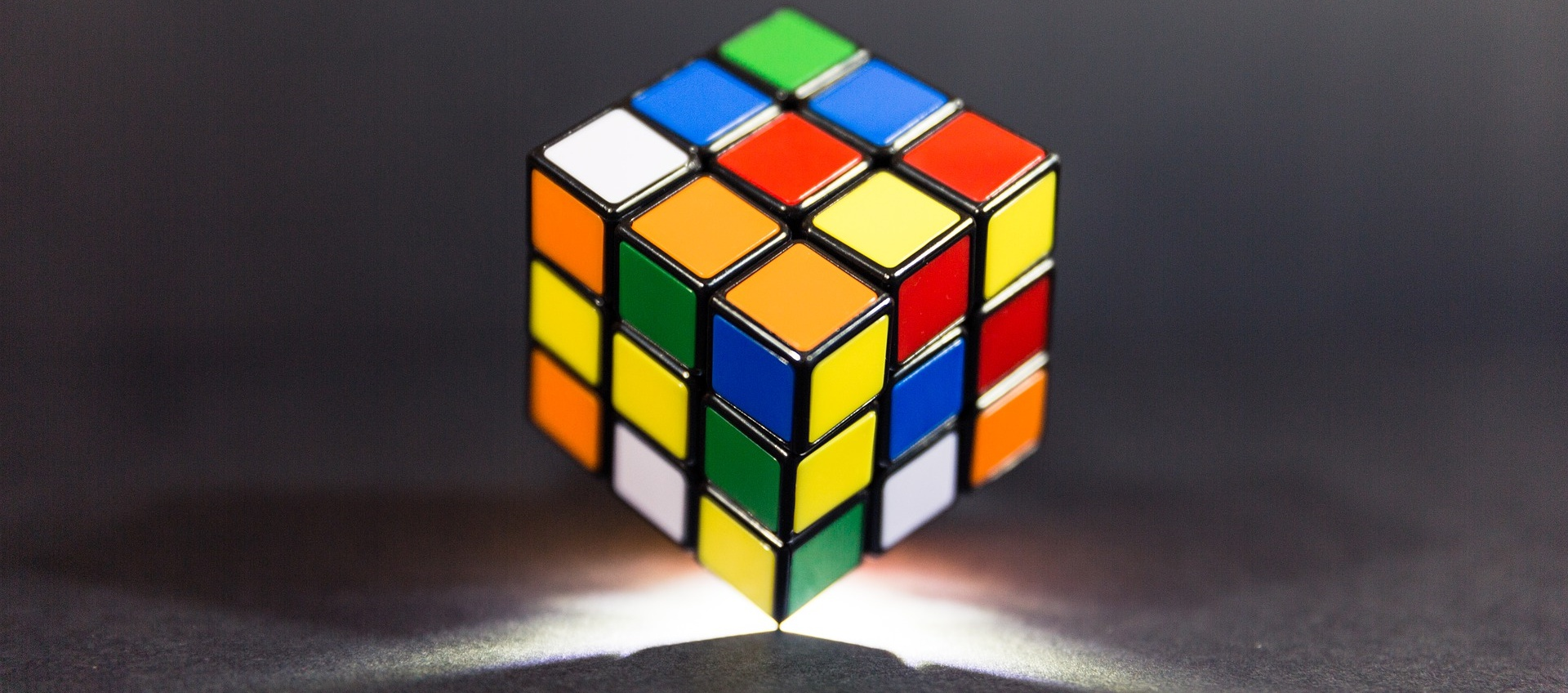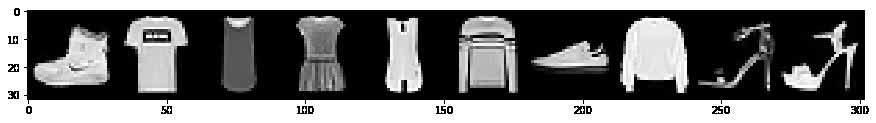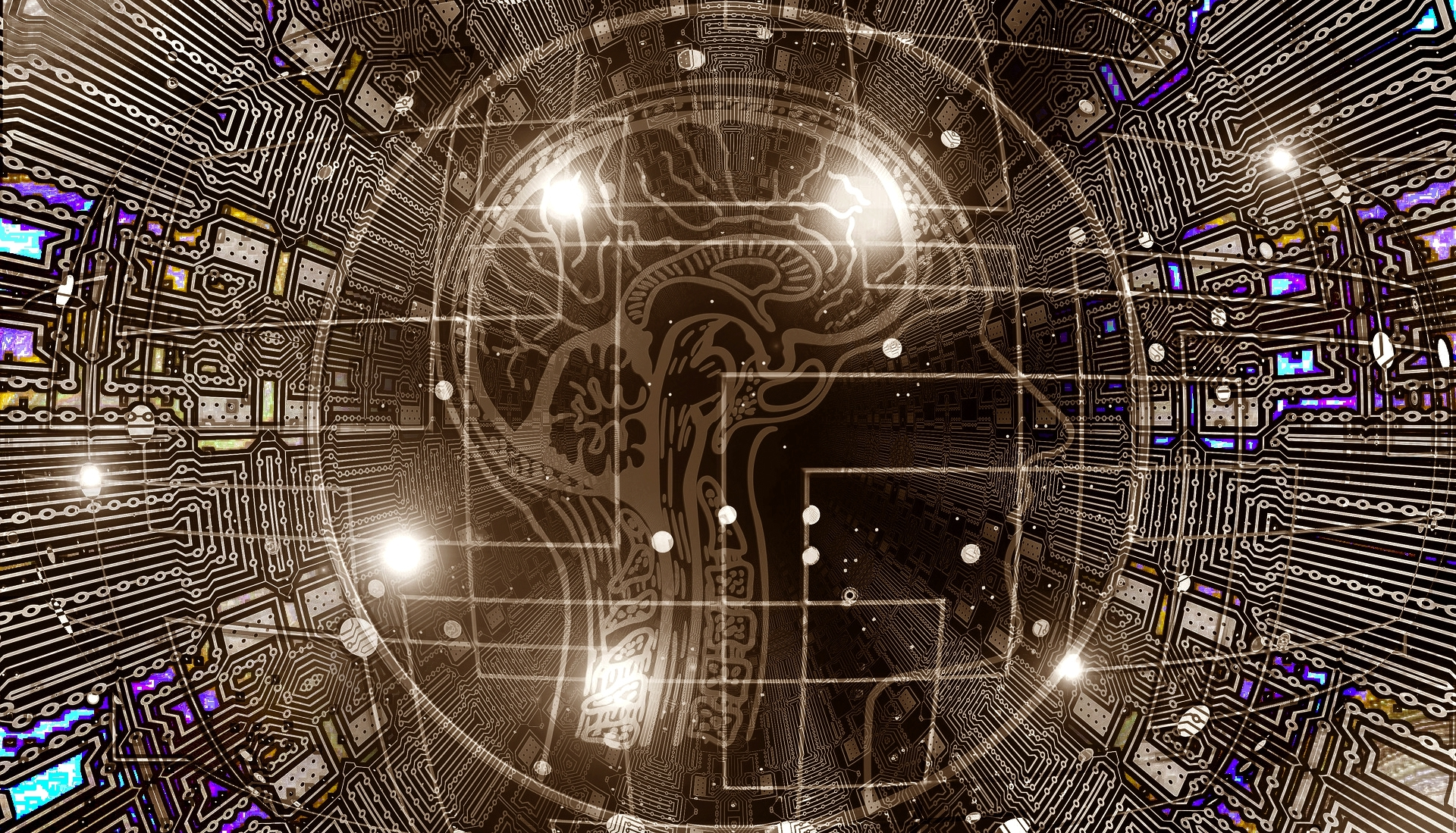# Neural Network Programming - Deep Learning with PyTorch

Deep Learning Course 3 of 5 - Level: Intermediate

## PyTorch Prerequisites - Syllabus for Neural Network Programming Course

### video

expand_more chevron_left

### text

expand_more chevron_left

### PyTorch prerequisites - Neural network programming series

What's going on everyone? Welcome to this series on neural network programming with PyTorch.In this post, we will look at the prerequisites needed to be best prepared. We'll get an overview of the series and a sneak peek at a project we'll be working on. This will give us a good idea about what we'll be learning, and what skills we'll have by the end of the series. Without further ado, let's jump right in with the details.

There are two primary prerequisites needed for this series:

1. Programming experience
2. Neural network experience

Let's look at what we need to know for both of these categories.

#### Programming experience

This neural network programming series will focus on programming neural networks using Python and PyTorch.Knowing Python beforehand is not necessary. However, understanding programming in general is a requirement. Any programming experience or exposure to concepts like variables, objects, and loops will be sufficient for successfully participating in this series.

#### Neural network experience

In this series, we'll be using PyTorch, and one of the things that we'll find about PyTorch itself is that it is a very thin deep learning neural network API for Python.This means that, from a programming perspective, we'll be very close to programming neural networks from scratch. For this reason, it will definitely be beneficial to be aware of neural network and deep learning fundamentals. It's not a requirement, but it's recommended to take the deep learning fundamentals series first.

### Neural Network Programming Series - Syllabus

To kick the series off, we have two parts. Let's look at the details of each part:

• Part 1: Tensors and Operations
• Section 1: Introducing PyTorch
• PyTorch Prerequisites - Neural Network Programming Series
• PyTorch Explained - Python Deep Learning Neural Network API
• PyTorch Install - Quick and Easy
• CUDA Explained - Why Deep Learning Uses GPUs
• Section 2: Introducing Tensors
• Tensors Explained - Data Structures of Deep Learning
• Rank, Axes, and Shape Explained - Tensors for Deep Learning
• CNN Tensor Shape Explained - CNNs and Feature Maps
• Section 3: PyTorch Tensors
• PyTorch Tensors Explained - Neural Network Programming
• Creating PyTorch Tensors for Deep Learning - Best Options
• Section 4: Tensor Operations
• Flatten, Reshape, and Squeeze Explained - Tensors for Deep Learning
• CNN Flatten Operation Visualized - Tensor Batch Processing
• Tensors for Deep Learning - Broadcasting and Element-wise Operations
• ArgMax and Reduction Ops - Tensors for Deep Learning
• Part 2: Neural Network Training
• Section 1: Data and Data Processing
• Importance of Data in Deep Learning - Fashion MNIST for AI
• Extract, Transform, Load (ETL) - Deep Learning Data Preparation
• PyTorch Datasets and DataLoaders - Training Set Exploration
• Section 2: Neural Networks and PyTorch Design
• Build PyTorch CNN - Object Oriented Neural Networks
• CNN Layers - Deep Neural Network Architecture
• CNN Weights - Learnable Parameters in Neural Networks
• Callable Neural Networks - Linear Layers in Depth
• How to Debug PyTorch Source Code - Debugging Setup
• CNN Forward Method - Deep Learning Implementation
• Forward Propagation Explained - Pass Image to PyTorch Neural Network
• Neural Network Batch Processing - Pass Image Batch to PyTorch CNN
• CNN Output Size Formula - Bonus Neural Network Debugging Session
• Section 3: Training Neural Networks
• CNN Training - Using a Single Batch
• CNN Training Loop - Using Multiple Epochs
• Building a Confusion Matrix - Analyzing Results Part 1
• Stack vs Concat - Deep Learning Tensor Ops
• Using TensorBoard with PyTorch - Analyzing Results Part 2
• Hyperparameter Experimenting - Training Neural Networks
• Section 4: Neural Network Experimentation
• Custom Code - Neural Network Experimentation Code
• Custom Code - Simultaneous Hyperparameter Testing
• On the GPU - Training Neural Networks with CUDA
• Data Normalization - Normalize a Dataset
• PyTorch DataLoader Source Code - Debugging Session
• PyTorch Sequential Models - Neural Networks Made Easy
• Batch Norm In PyTorch - Add Normalization To Conv Net Layers

#### Neural network programming: Part 1

Part one of the neural network programming series consists of two sections.

Section one will introduce PyTorch and its features. Importantly, we'll see why we should even use PyTorch in the first place. Stay tuned for that. It's a must see!

Additionally, we'll cover CUDA, a software platform for parallel computing on Nvidia GPUs. If you've ever wondered why deep learning uses GPUs in the first place, we'll be covering those details in the post on CUDA! This is also a must see!

Section two will be all about tensors, the data structures of deep learning. Having a strong understanding of tensors is essential for becoming a deep learning programming pro, so we'll be covering tensors in detail.We'll be using PyTorch for this, of course, but the concepts and operations we learn in this section are necessary for understanding neural networks in general and will apply for any deep learning framework.

#### Neural network programming: Part 2

Part two of the neural network programming series is where we'll kick off the first deep learning project we'll be building together. Part two is comprised of three sections.

The first section will cover data and data processing for deep learning in general and how it relates to our deep learning project. Since tenors are the data structures of deep learning, we'll be using all of the knowledge learned about tensors from part one. We'll introduce the Fashion-MNIST dataset that we'll be using to build a convolutional neural network for image classification.We'll see how PyTorch datasets and data loaders are used to streamline data preprocessing and the training process.

The second section of part two will be all about building neural networks. We'll be building a convolutional neural neural network using PyTorch. This is where we'll see that PyTorch is super close to building neural networks from scratch. This section is also where the deep learning fundamentals series will come in-handy most because we'll see the implementation of many concepts that are covered in that series.

The third section will show us how to train neural networks by constructing a training loop that optimizes the network's weights to fit our dataset. As we'll see, the training loop is built using an actual Python loop.

### Project preview: Training a CNN with PyTorch

Our first project will consist of the following components:

1. Python imports
2. Data: ETL with the PyTorch Dataset and DataLoader classes
3. Model: Convolutional neural network
4. Training: The training loop
5. Analytics: Using a confusion matrix

By the end of part two of the neural network programming series, we'll have a complete understanding of this project, and this will enable us to be strong users of PyTorch as well as give us a deeper understanding of deep learning and neural networks in general.

### Resources for getting started

The neural network programming series will have plenty of resources that can ensure your success.Here is a list:

Be sure to check back this blog post as any resource updates will be reflected here and not in the video. Also, if you haven't already, check out the deeplizard hivemind for exclusive perks and rewards and consider joining.

Deep learning and programming are both super powers that allow us humans to make the world a better place for all. We can now do more than just be intelligent. We can build intelligence.We hope you'll join us in building collective intelligence by taking this series. Let us hear from you at the end, and importantly along the way! Good luck! I'll see you in the series.

### quiz

expand_more chevron_leftDEEPLIZARD Message notifications

### resources

expand_more chevron_left

expand_more chevron_leftDEEPLIZARD Message notifications